# Example 2: Exploring the COS Function

This tutorial provides instructions to create this web-based Python app to plot the cos function for particular parameters provided by the user. The user is required to provide the parameters $A$ and $B$ in the equation $y=Acos(Bx)$ along with the limits $a$ and $b$ of the plot.

## Step 1: Info​

• You can fill in the required fields as shown in the following image.
• You can use the default Favicon Image.
• The Primary Image used is available here.## Step 2: Inputs​

Start by creating the input group: "Function Parameters" and two numerical input variables.

Edit the first input variable:

• Set the variable name to capitala.
• Set label to "A".
• Set the default value to 1
• Restrict the range between a min of -10 and a max of 10 with a step of 0.001.

Click on APPLY CHANGES to save your work: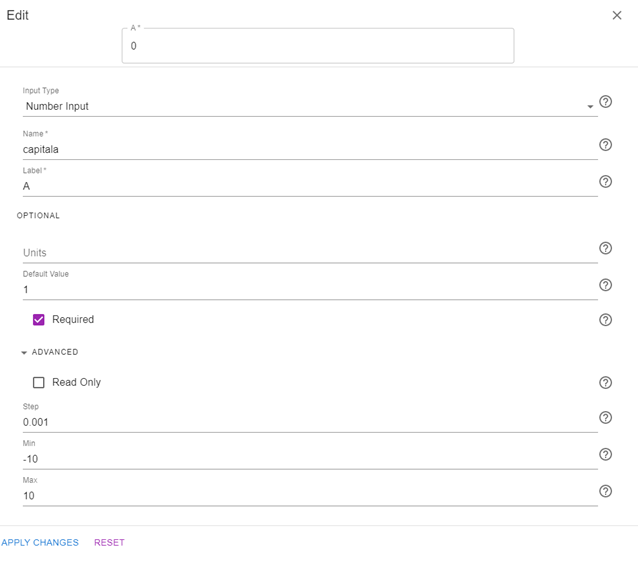Similarly, apply the changes shown in the following image to the second input.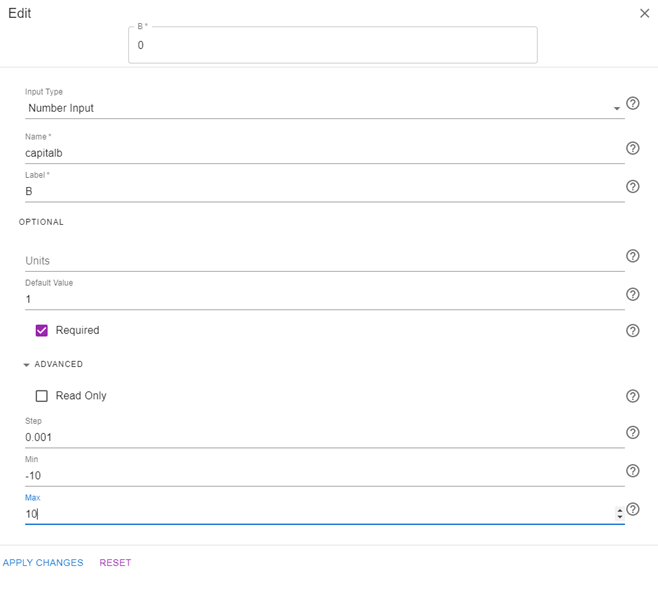You can also add a rich text input that clarifies to the user the form that the cosine equation uses and the limits of the parameters "A" and "B". To do so, click on the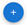button and drag in a RichText input. Hover over the recently created input and click on the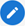button to edit it. Add the required text and use the latex equation generator if desired, as shown in the following image: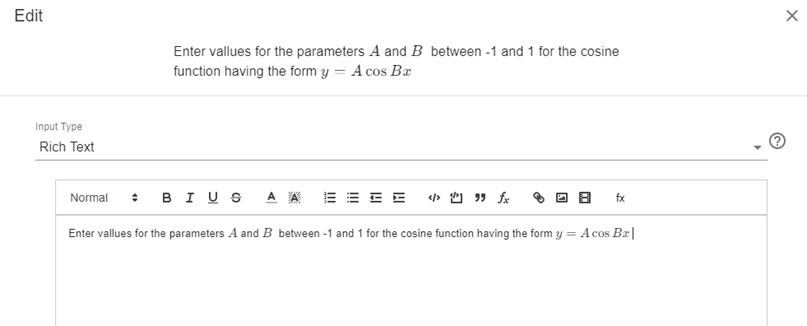You should now see the following inputs on your screen: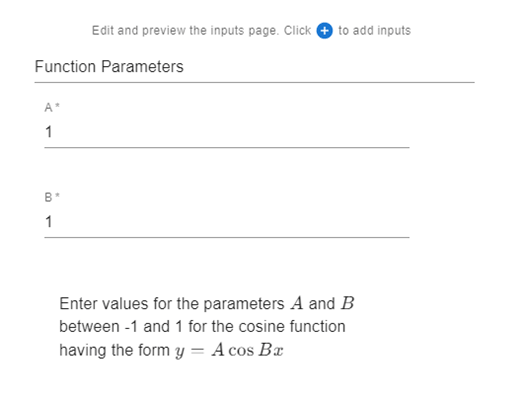Using your mouse, drag the rich text field and move it above the parameters "A" and "B":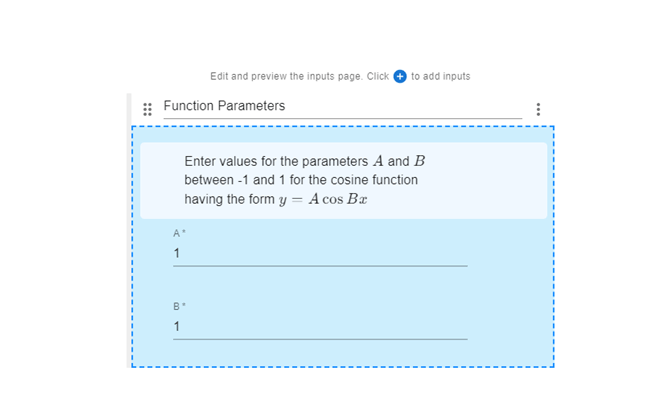Now, go ahead and create a new input group titled: "Plot Parameters". Create two inputs "a" and "b" to specify the left and right boundaries of the plot. Set their variable names as a and b respectively. Change their default values to -10 and 10 respectively. You should now see the following on your screen:## Step 3: Code​

As described in Generating Graphics, you need to first define the plt_show utility function which generates an embeddable image before defining the main function:

import matplotlib.pyplot as pltimport numpy as npimport base64import iodef plt_show(plt, width=500, dpi=100):    # Converts matplotlib plt to image data string    #   plt is the matplotlib pyplot or figure    #   width is the width of the graph image in pixels    #   dpi (dots per inch) is the resolution of the image    bytes = io.BytesIO()    plt.savefig(bytes, format='png', dpi=dpi)  # Save as png image    if hasattr(plt, "close"):        plt.close()    bytes.seek(0)    base64_string = "data:image/png;base64," + \        base64.b64encode(bytes.getvalue()).decode("utf-8")    return "<img src='" + base64_string + "' width='" + str(width) + "'>"def main(inputs):    # Code to generate a plot using matplot lib (plt)    # The following line uses the plt_show function to create an embeddable image    img = plt_show(plt)    return {"Image": img}

To access the inputs generated in the previous step, click on <> Input Variables, and copy each variable and paste it in a new variable assignment inside the main function:

def main(inputs):    A = inputs['capitala']    B = inputs['capitalb']    a = inputs['a']    b = inputs['b']    # Code to generate a plot using matplotlib (plt)    # The following line uses the plt_show function to create an embeddable image    img = plt_show(plt)    return {"Image": img}

You can now use matplotlib functions to generate the required plot:

def main(inputs):    A = inputs['capitala']    B = inputs['capitalb']    a = inputs['a']    b = inputs['b']    x = np.arange(a,b,0.01)    y = A*(np.cos(B*x))    plt.plot(x,y)    plt.xlabel("x")    plt.ylabel("y")    # The following line uses the plt_show function to create an embeddable image    img = plt_show(plt)    return {"Image": img}

## Step 4: Output​

You can simply output the image generated by the code: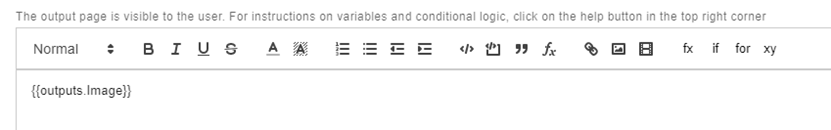## Step 5: Docs​

You can skip adding any documentation for this tutorial.

## Step 6: Preview​

In the preview section, confirm that the app works as expected: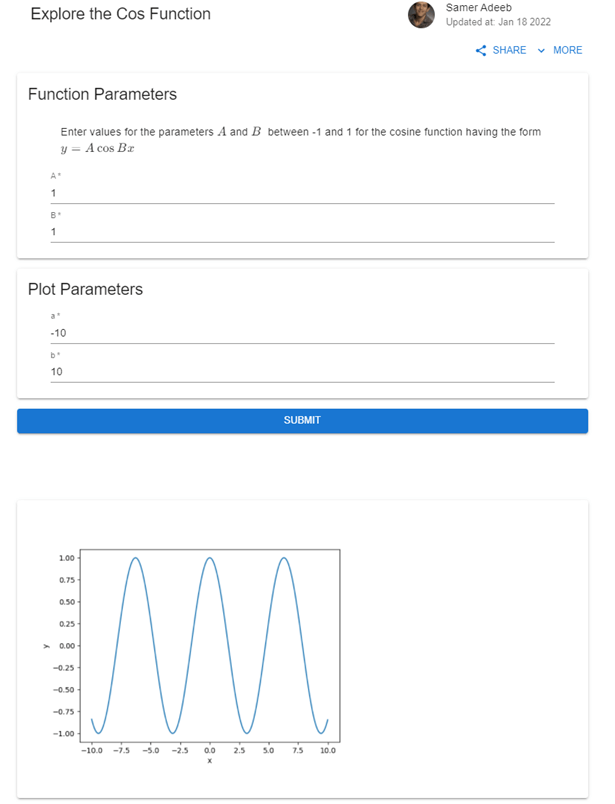Once ready, click on PUBLISH to publish the app on the web. This version of the app is available here:

https://www.mecsimcalc.com/app/5862152/explore_the_cos_function

Make sure to also check out the app that allows the user to input and plot any valid expression in x:

https://www.mecsimcalc.com/app/0153466/function_plot